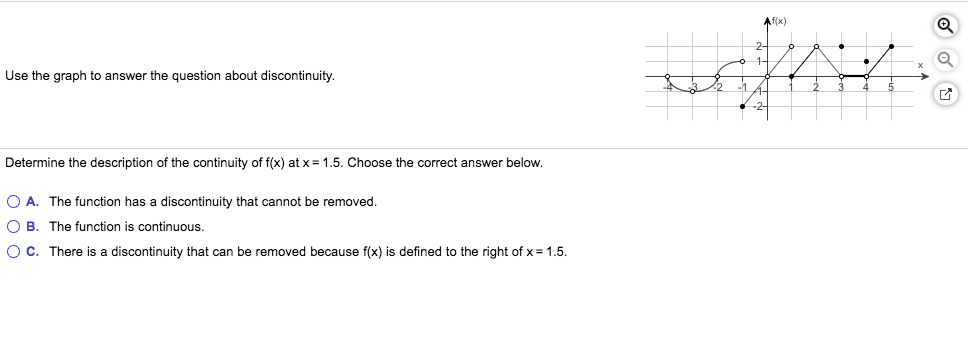Af(x)Use the graph to answer the question about discontinuity.Determine the description of the continuity of f(x) at x = 1.5. Choose the correct answer below.O A. The function has a discontinuity that cannot be removedO B. The function is continuous.O C. There is a discontinuity that can be removed because f(x) is defined to the right of x 1.5

Question

Can i get help with this problem step by step?help_outlineImage TranscriptioncloseAf(x) Use the graph to answer the question about discontinuity. Determine the description of the continuity of f(x) at x = 1.5. Choose the correct answer below. O A. The function has a discontinuity that cannot be removed O B. The function is continuous. O C. There is a discontinuity that can be removed because f(x) is defined to the right of x 1.5 fullscreen
Step 1

At x=-4,-3,-2,0 ,2,there are holes in the graph, it means the value of limit at these points exists but the value of the function is not defined at these points. so, x=-4,-3,-2,0,2,are points of discontinuity.

At x=-1 and x=1, the value of left hand limit is not equals to right hand limit, so limit of func...

Want to see the full answer?

See Solution

Want to see this answer and more?

Our solutions are written by experts, many with advanced degrees, and available 24/7

See Solution
Tagged in

Limits